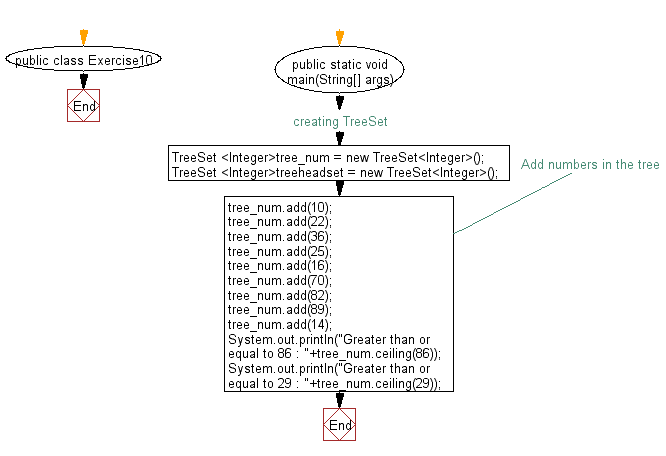﻿ Java: Find an element in a tree set greater, equal to other# Java Collection, TreeSet Exercises: Get the element in a tree set which is greater than or equal to the given element

## Java Collection, TreeSet Exercises: Exercise-10 with Solution

Write a Java program to get the element in a tree set which is greater than or equal to the given element.

Sample Solution:

Java Code:

``````import java.util.TreeSet;
import java.util.Iterator;

public class Exercise10 {
public static void main(String[] args) {
// creating TreeSet
TreeSet <Integer>tree_num = new TreeSet<Integer>();

// Add numbers in the tree

System.out.println("Greater than or equal to 86 : "+tree_num.ceiling(86));
System.out.println("Greater than or equal to 29 : "+tree_num.ceiling(29));
}
}
``````

Sample Output:

```Greater than or equal to 86 : 89
Greater than or equal to 29 : 36```

Flowchart:Java Code Editor:

What is the difficulty level of this exercise?

Test your Programming skills with w3resource's quiz.

﻿

## Java: Tips of the Day

getEnumMap

Converts to enum to Map where key is the name and value is Enum itself.

```public static <E extends Enum<E>> Map<String, E> getEnumMap(final Class<E> enumClass) {
return Arrays.stream(enumClass.getEnumConstants())
.collect(Collectors.toMap(Enum::name, Function.identity()));
}
```

Ref: https://bit.ly/3xXcFZt